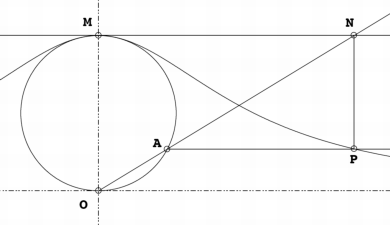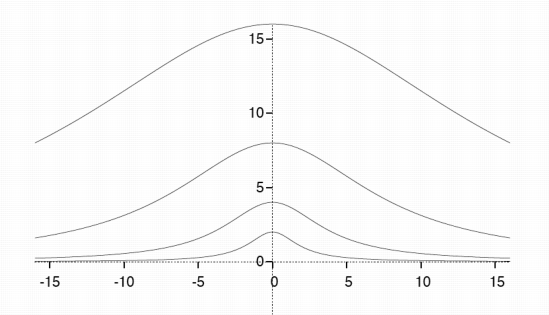### The witch of Agnesi

The first woman known as mathematichian was the italian Maria Gaetana Agnesi (1718-1799) and hermost famous work was Instituzioni analitiche ad uso della gioventù italiana (Analytical institutions for italian young people), in which she studied an old famous curve callde versiera, in anglosaxon worlds the witch of Agnesi. The name derives from a mistranslation by John Colson who intend the original italian therm averisera, that means versed sine curve, like avversiera, that in english became witch or wife of devil.
The curve was studied for the first time by Pierre de Fermat in 1630, and after by Guido Grandi in 1703 and in 1748 by Maria in her treatise. The curve was defined by:(commons)
Starting with a fixed circle, a point $O$ on the circle is chosen. For any other point $A$ on the circle, the secant line $OA$ is drawn. The point $M$ is diametrically opposite $O$. The line $OA$ intersects the tangent of $M$ at the point $N$. The line parallel to $OM$ through $N$, and the line perpendicular to $OM$ through $A$ intersect at $P$. As the point $A$ is varied, the path of $P$ is the witch.
Its cartesian equation is $y = \frac{8a^3}{x^2 + 4a^2}$ In 1918 Frederick H. Hodge proofed that the witch is generated from the following curve $a^2 ( x^2 - 2 (a+k) (2a - y))^2 = k^2x^2 (2ay - y^2)$ when $k$ goes to $\infty$.The Witch of Agnesi with parameters $a=1$, $a=2$, $a=4$, $a=8$
(commons)
Witch of Agnesi (Wikipedia)
Weisstein, Eric W. Witch of Agnesi. From MathWorld--A Wolfram Web Resource.
Hodge, F. (1918). Discussions: Relating to Generalizations of the Witch and the Cissoid The American Mathematical Monthly, 25 (5) DOI: 10.2307/2972650
Witch of Agnesi
Maria Gaetana Agnesi, biography on MacTutor
Applets:
The living witch of Agnesi
Witch of Agnesi on Wolfram Demonstrations Project